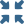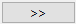# Create Linear Superposition of Results

View derived result that combines multiple load cases together.

Before you begin, you must have one or more linear structural, multi-loadcase or thermal analysis results.
Linear superposition is mathematically represented by the following equation:
Where,
${R}_{i}$
A vector of results (displacements, stresses, strains, and so on) for each individual load case.
${c}_{i}$
Scale factor.

Common usage examples are for structures with varying loads under operation. Not all loads are active at the same time, so a set of possible unit loads cases is created then combined later with varying scale factors to find the worst combination of loads.

The principle of superposition is applicable to linear analysis within a single design study. Non-linear analyses are not possible. In SimSolid, results superposition is supported for linear static and thermal analysis load cases only.

1. On the main toolbar, selectPost study.
2. Select either Structural superposition or Thermal superposition.
3. In the dialog, hold down Control + Shift and select the results to combine.
4. Click thebutton to combine analyses.
5. Click Solve.
All combined results will be located in the Post study branch of the Project Tree.

Structural linear superposition supports all post processing options supported by structural linear analysis. Thermal linear superposition supports all post processing options supported by thermal analysis.